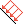# Pressure

The Pressureoption in the RocPlane Support menu allows you to specify a distributed force that is applied to the Slope Face and / or the Upper Face of the wedge. The Pressure is specified in terms of force per unit area and may represent:

• Support pressure (for example, rock bolts applied to the slope face of the wedge)

The Pressure option is the easiest way to model the effect of pattern bolting or distributed surcharge loads because the total force applied to the wedge is automatically calculated by RocPlane based on the wedge face area(s) and the pressure magnitude.

To use the Pressure option:

1. Select Pressure on the Support menu.
2. In the Pressure dialog, use the check boxes to select which face of the wedge you would like to apply the pressure to (the Slope Face or the Upper Face of the wedge, or both).
3. Enter an orientation (Angle).
NOTE: By default, the Angle of the pressure is normal to the face of the wedge (i.e., the Slope Face or the Upper Face). However, you may enter any orientation by entering the desired Angle. For example, if you are modeling a horizontal rock bolt pattern applied to the Slope Face, enter Angle = 0).
4. Enter a value for the Pressure (i.e., force per unit area of the wedge face).
EXAMPLE: If rock bolts with an individual capacity = 20 tonnes are installed on the Slope Face at a 2 meter by 2 meter spacing, the equivalent support Pressure equals 20 / (2 * 2) = 5 tonnes per square meter. This is the support Pressure to enter in the dialog.
5. Select either Active or Passive as the Model (for details, see How Bolts are Implemented in RocPlane.
6. Click Apply to run the analysis without closing the dialog or click OK to run the analysis and close the dialog.
You should see a change in the Factor of Safety.

NOTE: When you have defined a Pressure for a RocPlane model, the colour of the wedge face on which the pressure is applied is lightened to indicate that pressure is applied. In addition, an arrow in the same colour as the original wedge appears on the wedge face, indicating the direction of the applied Pressure.